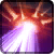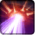# Unkontrolliert verstärkte ExplosionConditions

Used by

## Related effects, buffs and debuffs

Please click on an effect below to view its details.

• [does not expire]Unkontrolliert verstärkte Explosion
Diese Drohne macht sich bereit, einen verstärkten Energiestrahl auf ihr Ziel zu abzufeuern.

 Slot: Buff Duration: passive Tick rate: does not tick # occurrences: 0
• When channel ended
• [target override]

Only when the following conditions are met:

• <
• Is Target Caster
- Negated = (bool) true
AND
• If PRIMARY TARGET is alive
AND
• If PRIMARY TARGET has an effect with the tag tag.​abl.​operation.​iokath.​boss.​izax.​energy_drone.​is_energy_beam_sink
AND
• Unknown (84)
- Negated = (bool) false
- Block Identifier = (int) 1
>

Perform the following actions:

• Add effect #3 to TARGET from TARGET
• When channel ended

Only when the following conditions are met:

• Unknown (84)
- Negated = (bool) false
- Block Identifier = (int) 1

Perform the following actions:

• Add effect #2 to TARGET from TARGET
• [hidden] [1.5s]Effect #2

 Slot: Buff Duration: 1.5 Sek. Tick rate: does not tick # occurrences: 0 Conditions: Can only by called by other effects
• On Apply

Perform the following actions:

• Play appearance epp . operation . iokath . boss . izax . amplifier_drone . uncontrolled_beam_ray, dependent on calling effect
• Unknown (183)
- Unknown (303) = (bool) true
- Unknown (306) = (bool) false
- Unknown (430) = (bool) false
- Unknown (310) = (string) aoePositionOptionType_Entity
- Unknown (311) = (string) aoeTarget_Caster
- Unknown (313) = (string) aoeScalarType_LiteralValue
- Unknown (317) = (string) aoeScalarType_LiteralValue
- Unknown (321) = (string) aoeScalarType_LiteralValue
- Unknown (325) = (string) aoeOrientationOptionType_EntityFacing
- Unknown (326) = (string) aoeTarget_Caster
- Unknown (344) = (string) aoeCylinder
- Unknown (349) = (string)
- Unknown (353) = (string) aoeScalarType_LiteralValue
- Unknown (354) = (string) aoeScalarType_LiteralValue
- Unknown (355) = (string) aoeScalarType_LiteralValue
- Unknown (367) = (string) aoeScalarType_LiteralValue
- Unknown (375) = (string) aoeScalarType_LiteralValue
- Unknown (384) = (string)
- Unknown (386) = (string) aoeAbilityCaster_Caster
- Unknown (387) = (string) aoeAbilityTarget_Target
- Unknown (431) = (string) aoeRotation_None
- Ability Spec = (int) 16141003657213250520
- Unknown (307) = (int) 1
- Unknown (308) = (int) 1
- Tick Rate = (float) 0.5
- Unknown (304) = (float) 1
- Unknown (305) = (float) 1
- Unknown (314) = (float) 0
- Unknown (318) = (float) 0
- Unknown (322) = (float) 0
- Unknown (362) = (float) 0
- Unknown (363) = (float) 0
- Unknown (364) = (float) 0
- Unknown (368) = (float) 0.17
- Unknown (376) = (float) 12
- Unknown (390) = (float) 0
- Unknown (398) = (float) 0.1
• Add effect #5 to TARGET from TARGET
• [hidden] [1.5s]Effect #3

 Slot: Buff Duration: 1.5 Sek. Tick rate: does not tick # occurrences: 0 Tags: tag.​abl.​operation.​iokath.​boss.​izax.​amplifier_drone.​amplified_energy_beam Conditions: Can only by called by other effects
• On Apply

Perform the following actions:

• Unknown (183)
- Unknown (303) = (bool) true
- Unknown (306) = (bool) false
- Unknown (430) = (bool) false
- Unknown (310) = (string) aoePositionOptionType_Entity
- Unknown (311) = (string) aoeTarget_Caster
- Unknown (313) = (string) aoeScalarType_LiteralValue
- Unknown (317) = (string) aoeScalarType_LiteralValue
- Unknown (321) = (string) aoeScalarType_LiteralValue
- Unknown (325) = (string) aoeOrientationOptionType_DirectionBetweenEntities
- Unknown (328) = (string) aoeTarget_Caster
- Unknown (329) = (string) aoeTarget_Target
- Unknown (344) = (string) aoeCylinder
- Unknown (349) = (string)
- Unknown (353) = (string) aoeScalarType_LiteralValue
- Unknown (354) = (string) aoeScalarType_LiteralValue
- Unknown (355) = (string) aoeScalarType_LiteralValue
- Unknown (367) = (string) aoeScalarType_LiteralValue
- Unknown (375) = (string) aoeScalarType_LiteralValue
- Unknown (384) = (string)
- Unknown (386) = (string) aoeAbilityCaster_Caster
- Unknown (387) = (string) aoeAbilityTarget_Target
- Unknown (431) = (string) aoeRotation_None
- Unknown (506) = (string) aoeScaling_Distance
- Unknown (507) = (string) aoeTarget_Caster
- Unknown (508) = (string) aoeTarget_Target
- Ability Spec = (int) 16141003657213250520
- Unknown (307) = (int) 1
- Unknown (308) = (int) 1
- Tick Rate = (float) 0.5
- Unknown (304) = (float) 1
- Unknown (305) = (float) 1
- Unknown (314) = (float) 0
- Unknown (318) = (float) 0
- Unknown (322) = (float) 0
- Unknown (362) = (float) 0
- Unknown (363) = (float) 0
- Unknown (364) = (float) 0
- Unknown (368) = (float) 0.17
- Unknown (376) = (float) 12
- Unknown (390) = (float) 0
- Unknown (398) = (float) 0.1
• On Apply

Only when the following conditions are met:

• If PRIMARY TARGET has an effect with the tag tag.​abl.​operation.​iokath.​boss.​izax.​amplifier_drone.​overchargeable_object

Perform the following actions:

• Unknown (164)
- Ability Spec = (int) 16141105433838080837
- From Actor = (int) 3
- To Actor = (int) 3
• On Apply

Only when the following conditions are met:

• If PRIMARY TARGET does not have any effect with the tag tag.​abl.​operation.​iokath.​boss.​izax.​izax.​is_izax

Perform the following actions:

• Play appearance epp . operation . iokath . boss . izax . amplifier_drone . amplified_beam_instant_segment, dependent on calling effect
• On Apply

Only when the following conditions are met:

• <
• If PRIMARY TARGET has an effect with the tag tag.​abl.​operation.​iokath.​boss.​izax.​izax.​is_izax
AND
• Unknown (84)
- Negated = (bool) false
- Block Identifier = (int) 1
>

Perform the following actions:

• Unknown (164)
- Ability Spec = (int) 16141159505958336051
- From Actor = (int) 2
- To Actor = (int) 3
• On Apply

Only when the following conditions are met:

• <
• If PRIMARY TARGET has an effect with the tag tag.​abl.​operation.​iokath.​boss.​izax.​amplifier_drone.​energy_charge_level
AND
• Unknown (84)
- Negated = (bool) false
- Block Identifier = (int) 1
>

Perform the following actions:

• Add effect #4 to TARGET from TARGET
• [hidden] [1s]Effect #4

 Slot: Buff Duration: 1 Sek. Tick rate: does not tick # occurrences: 0 Conditions: Can only by called by other effects
• On Apply

Only when the following conditions are met:

• <
• If TARGET has at least 4 effects with the tag tag.​abl.​operation.​iokath.​boss.​izax.​amplifier_drone.​energy_charge_level
AND
• Unknown (84)
- Negated = (bool) false
- Block Identifier = (int) 1
>

Perform the following actions:

• Modify Charges
- Ability Spec = (int) 0
- Amount Max = (float) 5
- Amount Min = (float) 5
- Amount Percent = (float) 0
• Add effect #5 to TARGET from TARGET
• On Apply

Only when the following conditions are met:

• <
• If TARGET has at least 3 effects with the tag tag.​abl.​operation.​iokath.​boss.​izax.​amplifier_drone.​energy_charge_level
AND
• Unknown (84)
- Negated = (bool) false
- Block Identifier = (int) 1
>

Perform the following actions:

• Modify Charges
- Ability Spec = (int) 0
- Amount Max = (float) 4
- Amount Min = (float) 4
- Amount Percent = (float) 0
• Add effect #5 to TARGET from TARGET
• On Apply

Only when the following conditions are met:

• <
• If TARGET has at least 2 effects with the tag tag.​abl.​operation.​iokath.​boss.​izax.​amplifier_drone.​energy_charge_level
AND
• Unknown (84)
- Negated = (bool) false
- Block Identifier = (int) 1
>

Perform the following actions:

• Modify Charges
- Ability Spec = (int) 0
- Amount Max = (float) 3
- Amount Min = (float) 3
- Amount Percent = (float) 0
• Add effect #5 to TARGET from TARGET
• On Apply

Only when the following conditions are met:

• <
• If TARGET has an effect with the tag tag.​abl.​operation.​iokath.​boss.​izax.​amplifier_drone.​energy_charge_level
AND
• Unknown (84)
- Negated = (bool) false
- Block Identifier = (int) 1
>

Perform the following actions:

• Modify Charges
- Ability Spec = (int) 0
- Amount Max = (float) 2
- Amount Min = (float) 2
- Amount Percent = (float) 0
• Add effect #5 to TARGET from TARGET
• [hidden] [0.5s]Effect #5

 Slot: Buff Duration: 0.5 Sek. Tick rate: does not tick # occurrences: 0 Conditions: Can only by called by other effects
• On Apply

Perform the following actions:

• Modify Charges
- Ability Spec = (int) 0
- Amount Max = (float) -10
- Amount Min = (float) -10
- Amount Percent = (float) 0